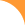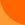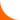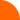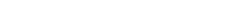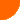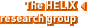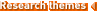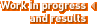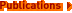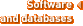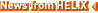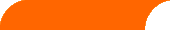Publications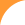Some approximation results for the maximum agreement forest problem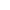Editors M. Goemans, K. Jansen, J. D. P. Rolim, L. Trevisan. In « Approximation, Randomization and Combinatorial Optimization: Algorithms and Techniques (APPROX & RANDOM 2001) », Lecture Notes in Computer Science, volume 2129, Springer-Verlag, 2001, pp.159-169.E. M. Rodrigues, M.-F. Sagot, Y. Wakabayashi.
 There are various techniques for reconstructing phylogenetic trees from data, and in this context the problem of determining how distant two such trees are from each other arises naturally. Various metrics (NNI, SPR, TBR) for measuring the distance between two phylogenies have been defined. Another way of comparing two trees T and U is to compute the so called maximum agreement forest of these trees. Informally, the number of components of an agreement forest tells how many edges need to be cut from each of T and U so that the resulting forests agree, after performing some forced edge contractions. This problem is known to be NP-hard. It was introduced by Hein et al. (1997), who presented an approximation algorithm for it, claimed to have approximation ratio 3. We present here a 3-approximation algorithm for this problem and show that the performance ratio of Hein's algorithm is 4.